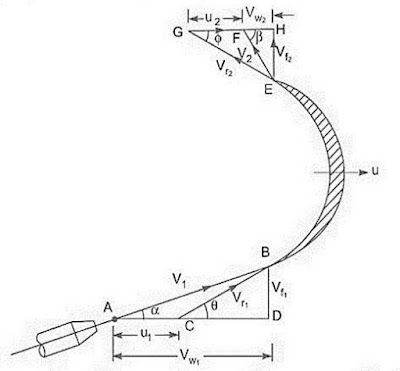# Velocity diagram or momentum equation - Ishwaranand

## Velocity diagramImage Source ~ Crafted With ©Ishwaranand - 2020 ~ Image by ©Ishwaranand

Fig. Velocity diagram
Let
• v1 = Velocity of the jet at inlet
• u1 = Velocity of the plate (vane) at the inlet.
• vr1 = Relative velocity from jet also plate at inlet
• α = Angle between the direction of the jet and direction of motion of the plate also called guide blade angle.
• θ = Angle made by the relative velocity (vr1) with the direction of motion at inlet also called vane angle at the inlet.
• vw1 and vf1 = The components of the velocity of the jet v1, in the direction of motion and perpendicular to the direction of motion of the vane respectively.
• vw1 = It is also known as the velocity of whirl at the inlet.
• vf1 = It is also known as the velocity of flow at inlet.
• v2 = Velocity of the jet, leaving the vane or velocity of jet at the outlet of the vane
• u2 = Velocity from the vane to outlet
• vr2 = Relative velocity of the jet by respect on the vane at outlet
• β =Angle made on the velocity v2 with each direction of motion from each vane at outlet
• ϕ = Angle made by the relative velocity vr2, with the direction of motion of the vane at the outlet and also called vane angle at outlet
• vw2 and vf2 = Components of the velocity v2, in the direction of motion of vane and perpendicular to the direction of motion of vane at the outlet.
• vw2 = It is also called the velocity of whirl at the outlet.
• vf2 = Velocity of flow at the outlet.
The triangles ABD and EGH are called the velocity triangles at inlet and outlet.
These velocity triangles are drawn as
• Velocity Triangle at Inlet: Take any point A and draw a line AB - v1 in magnitude and direction which means line AB makes an angle α with the horizontal line AD.
• Following, draw a line AC = u1 into magnitude. Join C to B.
• Then CB represents the relative velocity of the jet at the inlet.
• If the loss of energy at inlet due to impact is zero, then CB must be in the tangential direction to the vane at the inlet. Of B draw a vertical line BD in the descending direction to join the horizontal line AC produced by D.
Then
BD = Represent the velocity of flow to inlet = vf1
AD = Represent the velocity of whirl to inlet = vw1
BCD = Vane angle to inlet = θ
• Velocity Triangle at Outlet: If the vane surface is assumed to be very smooth, the loss of energy due to friction will be zero.
• The water will be gliding over the surface of the vane with a relative velocity equal to vr1 and will come out of the vane with a relative velocity vr2.
• This means that the relative velocity at outlet vr1 = vr2.
• And also the relative velocity at the outlet should be in a tangential direction to the vane at the outlet.
• Draw EG in the tangential direction of the vane at the outlet and cut EG = vr2. From G, draw a line GF in the direction of the vane at the outlet and equal to u2, the velocity of the vane at the outlet. Join EF. Then EF represents the absolute velocity of the jet at an outlet in magnitude and direction. Of E draw any vertical line EH to join the line GF produced on H.
Then,
FH = Velocity of whirl to outlet = vw2
ϕ = Angle of the vane at an outlet
β = Angle made with v2 with the direction of motion from vane at the outlet.

### Moment of momentum equation

Let
v1 = velocity at section 1
v2 = velocity at section 2
r1 = radius at section 1
r2 = radius at section 2
ρ = mass density
Q = rate of flow
Momentum of fluid/sec
= ρQ x v1
= (ρQ x v1) x r1
Moment of momentum per second at section 2
= (ρQ x v2) x r2
Rate of change of Moment of momentum per second
= Torque (T)
= ρQ (v2r2 – v1r1)